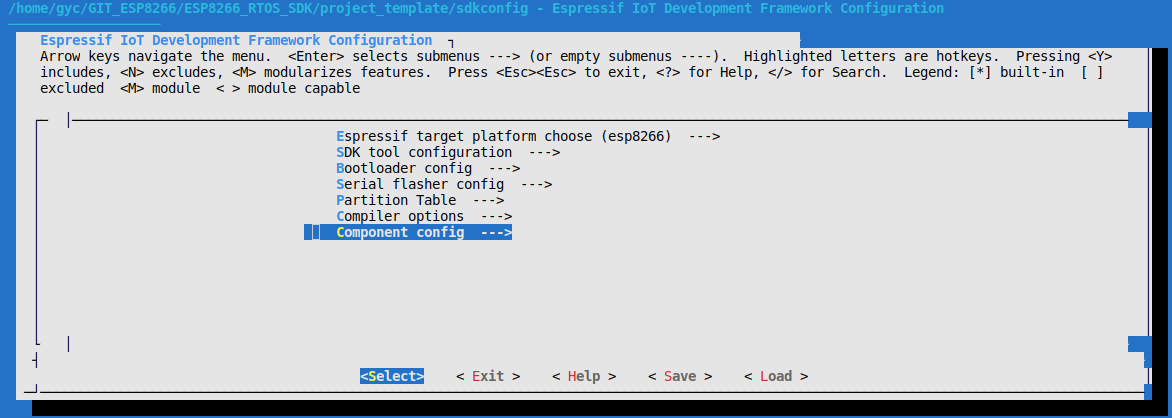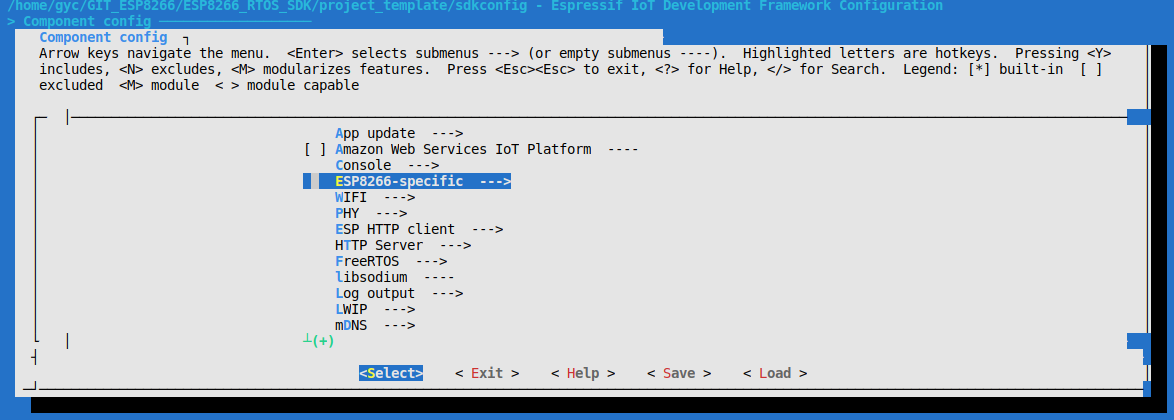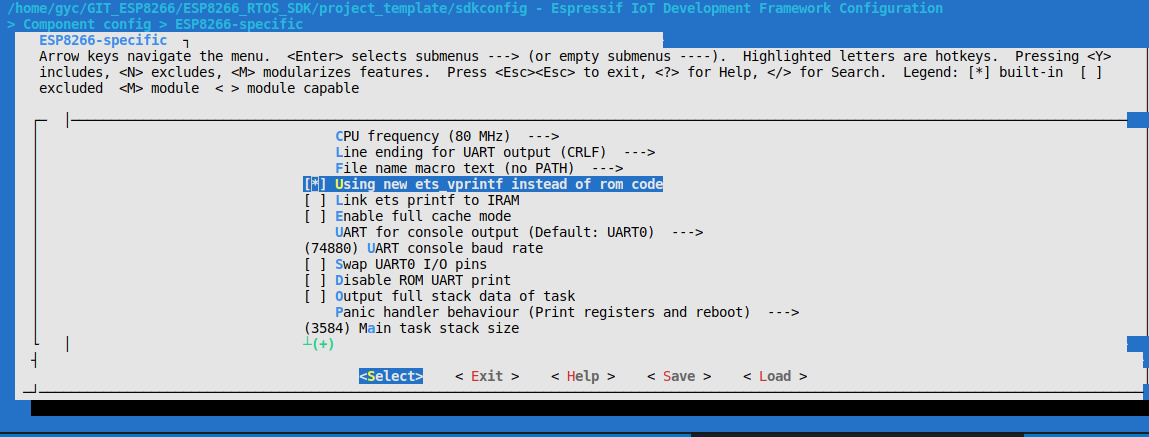# ① ESP8266 开发学习笔记_By_GYC 【更新 ets_printf 函数 使ESP_IDF 能够支持浮点数打印】

## 一、启用ets_printf函数

make menuconfig选择Component config选项卡  Enter进入 修改ESP8266工程宏定义配置选择 Using new ets_vprintf instead of rom code 选项 按下“空格”勾选。

## 二、修改ets_printf函数

case 'd':
attr.value.val32 = va_arg(va, int);
if (attr.value.val32 < 0) {
ets_putc('-');
attr.value.val32 = -attr.value.val32;
}
ets_printf_int(&attr, 10);
break;

由%d的 处理我发现需要在attr结构体里添加double型的变量，用来缓存变参数列表里的float或者double类型的变量。

原结构体类型是下面这样的：

typedef union _val_cache {
uint8_t         val8;
int32_t         val32;
uint32_t        val32u;
const char      *valcp;
} val_cache_t;

添加double类型的变量valfloat作为浮点数的缓存变量。新结构体类型如下：

typedef union _val_cache {
uint8_t         val8;
int32_t         val32;
uint32_t        val32u;
const char      *valcp;
double           valfloat;
} val_cache_t;

int ets_vprintf(const char *fmt, va_list va)

case 'f':

 case 'f':
attr.value.valfloat = va_arg(va, double);
if (attr.value.valfloat < 0) {
ets_putc('-');
attr.value.valfloat = -attr.value.valfloat;
}
ets_printf_float(&attr);
break;

#define FLOAT_decimals_MAX_NUM 9
#define VFLOAT_STR_MAX 20

static int ets_printf_float(val_attr_t * const attr)
{
char buf[VFLOAT_STR_MAX];
unsigned char offset = VFLOAT_STR_MAX;

int32_t integer=attr->value.valfloat;
double decimals = (attr->value.valfloat-integer);

if (attr->precision!=0) {
for (int i =0; i<attr->precision;i++) {
decimals=decimals*10.0;
}

integer=decimals;
if(decimals-integer>0.5)//末位四舍五入
integer++;

for (int i =0; i<attr->precision;i++) {
unsigned char c = integer % 10;
buf[--offset] = c + '0';
integer /= 10;
}
}
else
{
int i =0;
for (i =0; i<FLOAT_decimals_MAX_NUM;i++) {
decimals=decimals*10.0;
int digit=decimals;
if((decimals-(digit))==0.0)
{
i++;
break;
}
}
integer=decimals;
if(decimals-integer>0.5)
integer++;
for (; i>0;i--) {
unsigned char c = integer % 10;
buf[--offset] = c + '0';
integer /= 10;
}
}

buf[--offset] = '.';

integer=attr->value.valfloat;

if (integer != 0) {
for (; integer > 0; integer /= 10) {
unsigned char c = integer % 10;
buf[--offset] = c + '0';
}
} else
buf[--offset] = '0';

if (fill_num(attr)) {
char fill_data = isfill_0(attr) ? '0' : ' ';
unsigned char len = fill_num(attr) - (VFLOAT_STR_MAX - offset);
unsigned char left = fill_num(attr) > (VFLOAT_STR_MAX - offset) ? len : 0;

if (!isfill_left(attr)) {
ets_printf_ch_mutlti(fill_data, left);
}

ets_printf_buf(&buf[offset], VFLOAT_STR_MAX - offset);

if (isfill_left(attr)) {
fill_data = ' ';
ets_printf_ch_mutlti(fill_data, left);
}
} else {
ets_printf_buf(&buf[offset], VFLOAT_STR_MAX - offset);
}

return 0;
}


## 三、直接使用